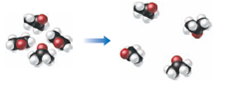×
Get Full Access to Introductory Chemistry - 5 Edition - Chapter 3 - Problem 115p
Get Full Access to Introductory Chemistry - 5 Edition - Chapter 3 - Problem 115p

×

# Solved: This molecular drawing shows images of acetone molecules before and after aISBN: 9780321910295 34

## Solution for problem 115P Chapter 3

Introductory Chemistry | 5th Edition

• Textbook Solutions
• 2901 Step-by-step solutions solved by professors and subject experts
• Get 24/7 help from StudySoup virtual teaching assistantsIntroductory Chemistry | 5th Edition

4 5 1 256 Reviews
23
0
Problem 115P

This molecular drawing shows images of acetone molecules before and after a change. Was the change chemical or physical?Step-by-Step Solution:
Step 1 of 3

Solution 115P

Here we can see that the after the process the molecules on the right and left sides are actually the same.The...

Step 2 of 3

Step 3 of 3

##### ISBN: 9780321910295

Since the solution to 115P from 3 chapter was answered, more than 335 students have viewed the full step-by-step answer. Introductory Chemistry was written by and is associated to the ISBN: 9780321910295. The full step-by-step solution to problem: 115P from chapter: 3 was answered by , our top Chemistry solution expert on 05/06/17, 06:45PM. This full solution covers the following key subjects: change, images, acetone, chemical, drawing. This expansive textbook survival guide covers 19 chapters, and 2046 solutions. This textbook survival guide was created for the textbook: Introductory Chemistry, edition: 5. The answer to “This molecular drawing shows images of acetone molecules before and after a change. Was the change chemical or physical?” is broken down into a number of easy to follow steps, and 19 words.

Unlock Textbook Solution